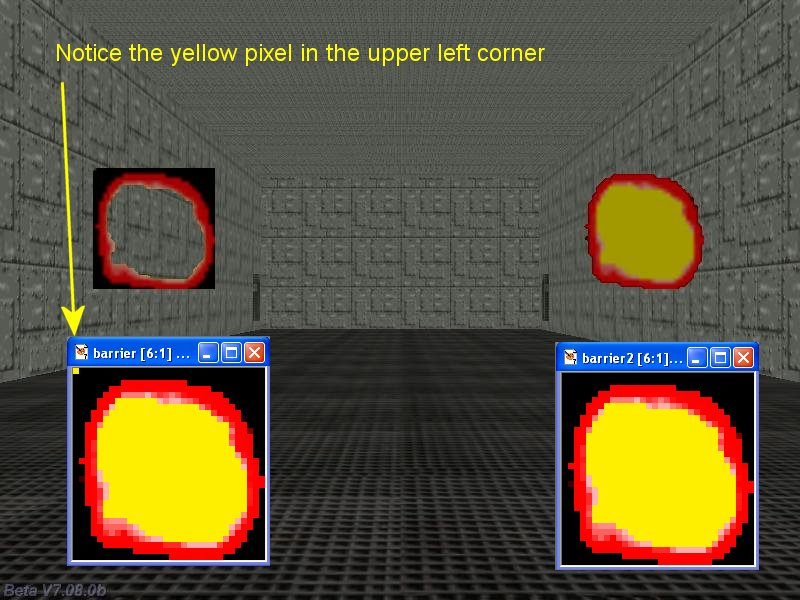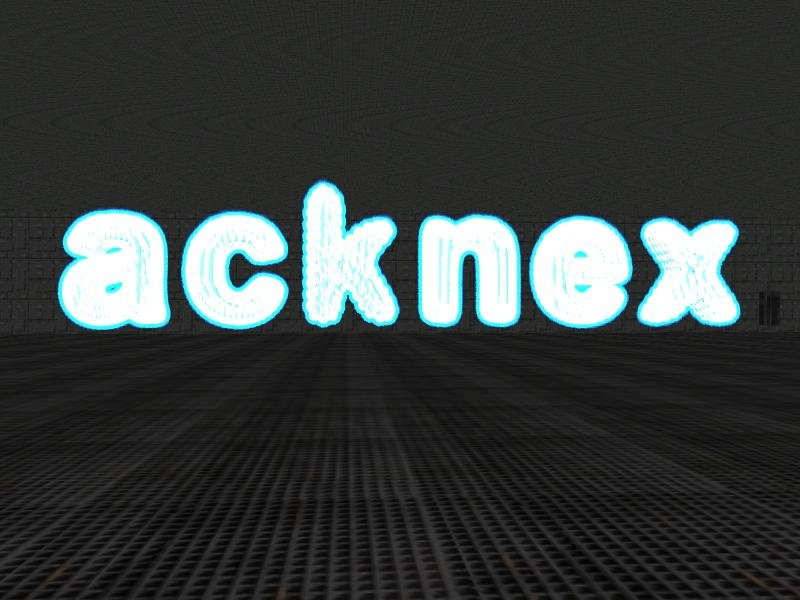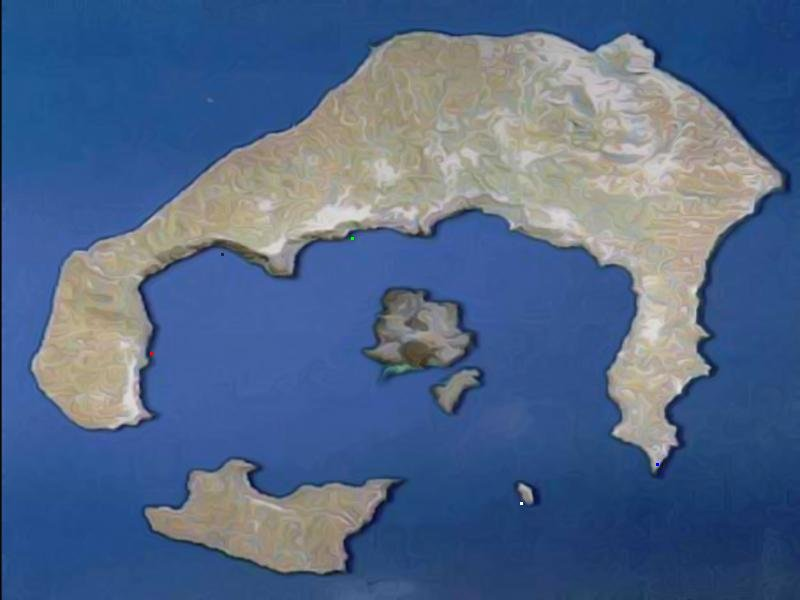Questions from the forum
 Q: Anyone got a snippet that makes a text appear when you touch something and makes it disappear when you move away? A: Here's an example:   TEXT* my_text = {        pos_x = 50;        pos_y = 30;        layer = 10;        string ("Please don't kill me!"); }     function i_am_touched() {        set(my_text, VISIBLE);         }   action coward_entity() {        while (!player) {wait (1);}        my.emask |= (ENABLE_IMPACT | ENABLE_ENTITY);        my.event = i_am_touched;        while (1)        {                if (vec_dist (player.x, my.x) > 200)                {                        reset(my_text, VISIBLE);                }                wait (1);        } }     Q: I would need a script that makes the camera follow the mouse pointer gently, but stops the camera when the pointer is close to the center of the screen. A: There you go.   BMAP* arrow_pcx = "arrow.pcx";   function mouse_startup() {         mouse_mode = 1;        mouse_map = arrow_pcx;        while (1)        {           vec_set(mouse_pos, mouse_cursor);      wait(1);        } }   function camera_startup() {        VECTOR* offset;        while (1)        {                offset.x = mouse_pos.x - screen_size.x / 2;                offset.y = mouse_pos.y - screen_size.y / 2;                // allow a zone of 60 pixels in the center of the screen where the camera doesn't follow the mouse pointer                if (abs(offset.x) > 30)                {                        camera.pan -= 0.01 * offset.x * time_step; // 0.01 gives the rotation speed                }                if (abs(offset.y) > 30)                {                        camera.tilt -= 0.01 * offset.y * time_step;                }                wait (1);        } }     Q: I would need a 3D arrow that points downwards, moving up and down, in order to show the player the following task. A: Create a 3D arrow model that points downwards and then attach it this snippet.   action my_arrow() {        while (1)        {                // find the best combination by playing with 3 (amplitude) and 15 (speed)                my.z += 3 * sin(total_ticks * 15) * time_step;                wait (1);        } }     Q: I want to play footstep sounds in time with my walking animation, I was wondering if it's possible to find out what frame is being played in the current animation and then play a sound. A: First of all, open your model in Med; I will use the guard.mdl model as an example. This model has four "walk" frames, with "walk2" and "walk4" looking good for us. This means that the animation percentages that should trigger the sound steps are at the middle of the "walk" cycle (50%) and close to the end of the "walk" cycle; let's choose 95% for the end value because 100% might not be reached that often. And now take a look at the code that makes it happen.   SOUND* step_wav = "step.wav";   // the action doesn't include a c_move line of code in order to allow you to see the footsteps clearly action footstep_guy() {        var anim_percentage;        var sounded_once;        while (1)        {                ent_animate(my, "walk", anim_percentage, ANM_CYCLE);                anim_percentage += 3 * time_step; // "3" controls the animation speed                anim_percentage %= 100;                if (anim_percentage < 10)                {                        sounded_once = 1;                }                if ((anim_percentage > 50) && (sounded_once == 1)) // play the first footstep sound at 50%                {                        sounded_once = 2;                        ent_playsound(my, step_wav, 200);                }                if ((anim_percentage > 95) && (sounded_once == 2)) // play the second footstep sound at 95%                {                        sounded_once = 3;                        ent_playsound(my, step_wav, 200);                }                wait (1);        } }     Q: I want to place several sprites in the game but they have a background that I don't want to be visible. How can I tell the engine to ignore a certain color of a bitmap? A: Paste the code below in your project and use tga textures without alpha channels for your sprites. The color that is used by the pixel in the upper left corner of the bitmap will be ignored, just like in the example below.   function set_transparency_startup() {        // use the color of the pixel in the upper left corner of the bitmap for transparency        d3d_autotransparency = 1; }Q: I want the closest camera to be set and look at the player as soon as the player approaches it. A: Here's an updated version of my "Resident Evil" camera snippet from Aum4.   var camera_distance; // up to 50 cameras var temp_counter = 0; // array index var winner = 3000; // default distance between the camera and the player var found_camera = 1; // index for the active camera   function cameras_startup() {        while (1)        {                temp_counter += 1;                temp_counter %= 50;                if ((camera_distance[temp_counter] < winner) && (camera_distance[temp_counter] > 0))                {                        winner = camera_distance[temp_counter];                        found_camera = temp_counter;                }                else                {                        winner = camera_distance[found_camera];                }                wait (1);        }         }   // set skill1 to 1 for the first camera, skill1 = 2 for the second camera and so on action recam() // attach this action to your camera entities {        var temp;        while (!player) {wait(1);}        set (my, INVISIBLE);        set (my, PASSABLE);        camera.tilt = my.tilt;        camera.roll = my.roll;        if (my.skill1 == 0) // the player has forgotten to set skill1 for one of the cameras?        {                beep(); // then beep twice and then shut down the engine                beep();                sys_exit(NULL);        }        while (1)        {                camera_distance[my.skill1] = vec_dist(my.x, player.x);                if (winner > camera_distance[my.skill1] - 50) // hysteresis                {                                camera.x = my.x;                        camera.y = my.y;                        camera.z = my.z;                        vec_set(temp, player.x);                        vec_sub(temp, my.x);                        vec_to_angle(camera.pan, temp);                }                wait (1);        } }             Q: I'm wondering if you can help me creating a crosshair that is controlled with the arrow keys, while the player can be moved using the WSAD keys. A: Here's an example.   BMAP* cross_tga = "cross.tga";   PANEL* crosshair_pan = {        bmap = cross_tga;        pos_x = 400;        pos_y = 300;        flags = VISIBLE; }   function crosshair_startup() // centers the crosshair on the screen regardless of the video resolution {        while (1)        {                crosshair_pan.pos_x = (screen_size.x - bmap_width(cross_tga)) / 2;                crosshair_pan.pos_y = (screen_size.y - bmap_height(cross_tga)) / 2;                wait (1);        } }   action players_code() {        player = my; // I'm the player        set (my, INVISIBLE); // no need to see player's model in 1st person mode        while (1)        {                // move the player using the "W", "S", "A" and "D" keys; "10" = movement speed, "6" = strafing speed                c_move (my, vector(10 * (key_w - key_s) * time_step, 6 * (key_a - key_d) * time_step, 0), nullvector, GLIDE);                if (key_space) // if the player presses the space key fire a bullet                {                        // create your own function that fires bullets or get it from one of the Aum workshops                }                vec_set (camera.x, player.x); // use player's x and y for the camera                camera.z += 30; // place the camera 30 quants above the player on the z axis (approximate eye level)                camera.pan -= 5 * key_force.x * time_step; // rotate the camera around using the arrow keys                camera.tilt += 3 * key_force.y * time_step; // do this on the x and y axis                player.pan = camera.pan; // the camera and the player have the same pan angle                wait (1);        } }     Q: How can I create a particle glowing effect, surrounding a 3D model? A: Use the following effect.   BMAP* particle_tga = "particle.tga";   function init_startup() {        max_particles = 50000; }   function fade_particle(PARTICLE *p) {        p.alpha -= 50 * time_step;        if (p.alpha < 0)                p.lifespan = 0; }   function particle_function(PARTICLE *p) {        vec_add (p.vel_x, vector(0, 0, 5 + random(1)));        set(p, MOVE | BRIGHT);        p.alpha = 30 + random(35);        p.bmap = particle_tga;        p.size = 30;        p.lifespan = 30;        p.event = fade_particle; }   action glowing_models() {        var particle_pos;        while (1)        {                my.skill1 = 0;                while (my.skill1 < ent_vertices (my)) // go through all the vertices of the model                {                        my.skill1 += 1;                        vec_for_vertex(particle_pos, my, my.skill1);                        effect(particle_function, 1, particle_pos, nullvector);                }                wait (1);        } }Q: Is there a simple way to display several tiny colored dots on top of a map? My best try is to use lots of 1x1 pixel panels. A: There you go.   var map_visible = 0;   BMAP* map_tga = "map.tga";   PANEL* map_pan = {        bmap = map_tga;          layer = 20;                 pos_x = 0;          pos_y = 0; }   function toggle_it() {        map_visible += 1;        map_visible %= 2;        if (map_visible)        {                set (map_pan, VISIBLE);        }                        else        {                reset (map_pan, VISIBLE);        } }   function toggle_map_startup() {        on_m = toggle_it; }   function draw_dots_startup() {        draw_textmode("Arial", 1, 20, 100); // set the font, its type and size        var alpha_factor = 0;        while(1)        {                  if (map_visible)                {                        draw_text(".", 150, 340, vector(0, 0, 255)); // plot a red pixel at 150, 340                        draw_text(".", 350, 225, vector(0, 255, 0)); // plot a green pixel at 350, 225                        draw_text(".", 655, 450, vector(255, 0, 0)); // plot a blue pixel at 655, 450                        draw_text(".", 220, 240, vector(32, 32, 32)); // plot a dark pixel at 220, 240                        draw_text(".", 520, 490, vector(255, 255, 255)); // plot a white pixel at 520, 490                }                wait(1);        } }Q: I have used WED to design the first level of my game. I build the level but when I run it none of the sprites are visible. What is wrong? A: Two things can cause the problem: - You are using tga sprites without alpha (transparency) channels. The newer versions of the engine need this. - The camera is penetrating a wall. Press the zero key to free it and then use the arrow keys to move it somewhere else or (even better) get and use a player / camera snippet from Aum.